GATE  >  GATE Numerical Computation Aptitude (Quant) Questions - 1

# GATE Numerical Computation Aptitude (Quant) Questions - 1

Test Description

## 10 Questions MCQ Test General Aptitude for GATE | GATE Numerical Computation Aptitude (Quant) Questions - 1

GATE Numerical Computation Aptitude (Quant) Questions - 1 for GATE 2023 is part of General Aptitude for GATE preparation. The GATE Numerical Computation Aptitude (Quant) Questions - 1 questions and answers have been prepared according to the GATE exam syllabus.The GATE Numerical Computation Aptitude (Quant) Questions - 1 MCQs are made for GATE 2023 Exam. Find important definitions, questions, notes, meanings, examples, exercises, MCQs and online tests for GATE Numerical Computation Aptitude (Quant) Questions - 1 below.
Solutions of GATE Numerical Computation Aptitude (Quant) Questions - 1 questions in English are available as part of our General Aptitude for GATE for GATE & GATE Numerical Computation Aptitude (Quant) Questions - 1 solutions in Hindi for General Aptitude for GATE course. Download more important topics, notes, lectures and mock test series for GATE Exam by signing up for free. Attempt GATE Numerical Computation Aptitude (Quant) Questions - 1 | 10 questions in 15 minutes | Mock test for GATE preparation | Free important questions MCQ to study General Aptitude for GATE for GATE Exam | Download free PDF with solutions
 1 Crore+ students have signed up on EduRev. Have you?
GATE Numerical Computation Aptitude (Quant) Questions - 1 - Question 1

### Operators □ , ◊ and → are defined by: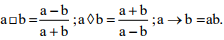Find the value (66 □ 6) → (66◊6).

GATE Numerical Computation Aptitude (Quant) Questions - 1 - Question 2

### The velocity V of a vehicle along a straight line is measured in m/s and plotted as shown with respect to time in seconds. At the end of the 7 seconds, how much will the odometer reading increase by (in m)?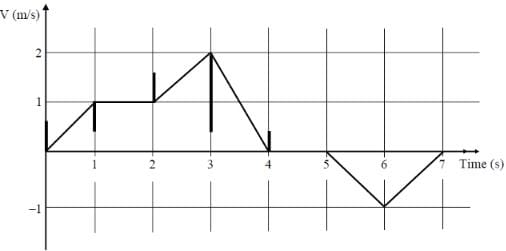Detailed Solution for GATE Numerical Computation Aptitude (Quant) Questions - 1 - Question 2

The odometer reading increases from starting point to end because odometer measures distance not displacement.
So, all the areas should be counted postive only.
Area of the given diagram = Odometer reading
Area of the velocity and time graph per second
1st sec triangle = 1/2 * 1 * 1= 1/2
2nd sec square = 1 × 1 = 1
3rd sec square + triangle = 1 × 1 + 1/2 × 1 × 1 = 3/2
4th sec triangle =1/2 * 1 * 2 = 1
5th sec straight line = 0
6th sec triangle = 1/2 * 1 * 1 = 1/2
7th sec triangle = 1/2 * 1 * 1 = 1/2
Total Odometer reading at 7 seconds = 1/2 + 1 + 3/2 + 1 + 0 + 1/2 + 1/2 = 5m

GATE Numerical Computation Aptitude (Quant) Questions - 1 - Question 3

### log tan 1o + log tan 2o +.....+ log tan 89o is _____.

Detailed Solution for GATE Numerical Computation Aptitude (Quant) Questions - 1 - Question 3

As per trigonometric properties

tan θ = cot (90 - θ) and tan θ . cot θ = 1

Thus, tan 89° = cot (90 -89° ) =  cot 1°

Similarly, tan 88° = cot 2°, tan 87° = cot 3° ans so on...

Also from Logarith properties

log m +log n = log (m × n)

Thus, log tan 1° + log tan 89° = log (tan1° × tan89°)

= log (tan1° × cot1°)

= log 1 = 0

similarly, log tan 2° + log tan 88° = log (tan1° × cot1°) = log 1 = 0

Thus, log tan1° +log tan2° +……..+log tan 89° = 0

GATE Numerical Computation Aptitude (Quant) Questions - 1 - Question 4

An electric bus has onboard instruments that report the total electricity consumed since the start of the trip as well as the total distance covered. During a single day of operation, the bust travels on stretches M, N, O and P, in that order. The cumulative distances traveled and the corresponding electricity consumption are shown in the Table below: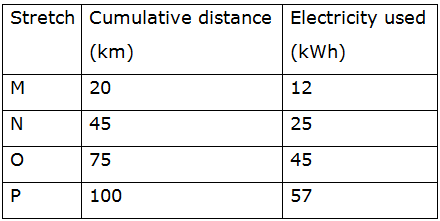Q. The stretch where the electricity consumption per km is minimum is

Detailed Solution for GATE Numerical Computation Aptitude (Quant) Questions - 1 - Question 4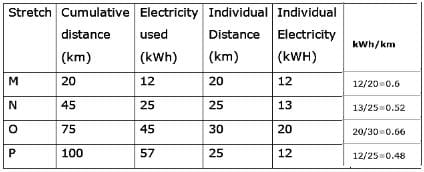GATE Numerical Computation Aptitude (Quant) Questions - 1 - Question 5

If a2 + b2 + c2 = 1, then ab + ac + bc lies in the interval

Detailed Solution for GATE Numerical Computation Aptitude (Quant) Questions - 1 - Question 5

Concept:

1. (a - b)2 = a2 - 2ab + b2

2. (a + b + c)2 = a2 + b2 + c2 + 2(ab + bc + ca)

Where, (a + b + c)2 > 0

If a, b & c ∀ 0, then

(a + b + c)2  = 0

Calculation:

Given that,

a2 + b2 + c2 = 1   ----(1)

We know that, (a + b + c)2 = a2 + b2 + c2 + 2(ab + bc + ca)

⇒ (ab + bc + ca) ≥ -1/2

Again  ( b − c )2 + ( c − a )2 + ( a − b )2 ≥ 0

⇒ bc + ca + ab ≤ a2 + b2 + c2 = 1

Hence  − 1/2 ≤ bc + ca + ab ≤ 1

GATE Numerical Computation Aptitude (Quant) Questions - 1 - Question 6

The Venn diagram shows the preference of the student population for leisure activities.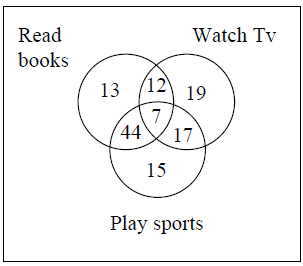Q. From the data given, the number of students who like to read books or play sports is_____

Detailed Solution for GATE Numerical Computation Aptitude (Quant) Questions - 1 - Question 6

From Venn diagram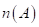= no of persons reading books =13 +44 +12 +7 = 76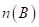= no of persons playing sports=15 + 44 + 7 +17 = 83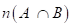=51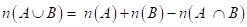=76+83-51=108
Another Approach
Number of students who like to read books or play sports will be the sum of students who belong to both sets
= 13 + 12 + 44 + 7 + 17 + 15 = 108.

GATE Numerical Computation Aptitude (Quant) Questions - 1 - Question 7

If x > y > 1, which of the following must be true?
(i) ln x > ln y
(ii) ex > ey
(iii) yx > xy
(iv) cos x > cos y

Detailed Solution for GATE Numerical Computation Aptitude (Quant) Questions - 1 - Question 7

From the logarithmic property,

when x > y

ln x > ln y if x and y both greater than 1.

and x > y

ln x < ln y, if both x and y are less than 1.

but when x > y

ex > ey  for any value of x and y.

but when x > y, x2 > y2

also cos x < cos y.

GATE Numerical Computation Aptitude (Quant) Questions - 1 - Question 8

From a circular sheet of paper of radius 30cm, a sector of 10% area is removed. If the remaining part is used to make a conical surface, then the ratio of the radius and height of the cone is ________.

Detailed Solution for GATE Numerical Computation Aptitude (Quant) Questions - 1 - Question 8

Area of Circle= πr2 = π.(30)2

Remaining Area = 0.9π(30)2 = 810π

Then Lateral Surface Area = πrl

Now 810π = π.rl where l = 30cm

810= r x 30

r = 27

h = √171

so r/h = 2.064

GATE Numerical Computation Aptitude (Quant) Questions - 1 - Question 9

A tiger is 50 leaps of its own behind a deer. The tiger takes 5 leaps per minute to the deer’s 4. If the tiger and the deer cover 8 meters and 5 meters per leap respectively. What distance in metres will be tiger have to run before it catches the deer?

Detailed Solution for GATE Numerical Computation Aptitude (Quant) Questions - 1 - Question 9

From The Question

A tiger is 50 leaps of its own from a deer.

Tiger takes 5 leaps per minute.

Deer takes 4 leaps per minute.

Tiger covers 8 meters per leap.

Deer covers 5 meters per leap.

Therefore, the tiger covers 5 x 8 = 40 meters/minute.
The deer covers 4 x 5 = 20 meters/minute.
Hence, the relative speed is 20 meters/minute.
The tiger is behind the deer by 50 x 8 = 400 meters.
Therefore, the time taken = distance/speed = 400/20 = 20 minutes.
Therefore, in the 20 minutes, the tiger will cover 20 x 40 = 800 meters.

GATE Numerical Computation Aptitude (Quant) Questions - 1 - Question 10

The statistics of runs scored in a series by four batsmen are provided in the following table: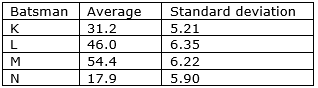Q. Who is the most consistent batsman of these four?

Detailed Solution for GATE Numerical Computation Aptitude (Quant) Questions - 1 - Question 10

Average only gives the mean value, Standard Deviation gives how close to mean value (consistency) of a sample population distribution.
A standard deviation close to 0 means very close to mean value of a distribution.
Here K has the lowest SD (5.21)

## General Aptitude for GATE

73 videos|86 docs|108 tests
 Use Code STAYHOME200 and get INR 200 additional OFF Use Coupon Code
Information about GATE Numerical Computation Aptitude (Quant) Questions - 1 Page
In this test you can find the Exam questions for GATE Numerical Computation Aptitude (Quant) Questions - 1 solved & explained in the simplest way possible. Besides giving Questions and answers for GATE Numerical Computation Aptitude (Quant) Questions - 1, EduRev gives you an ample number of Online tests for practice

## General Aptitude for GATE

73 videos|86 docs|108 tests